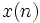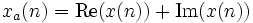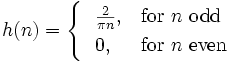# Difference between revisions of "Contributions:HilbertFilter"

## Synopsis

This filter computes the envelope or the phase of a signal using Hilbert transform. The discrete input signal$x(n)$ is first transformed to its analytic representation (i.e., analytic signal), which is composed of a real and an imaginary part.$x_{a}(n) = \operatorname{Re}(x(n)) + \operatorname{Im}(x(n))$

The real part is the same input signal, and the imaginary part is the Hilbert transform of the input signal. The Hilbert transform was implemented as the convolution of the input signal x(n) with the FIR filter h(n). To get a good approximation of the Hilbert transform, n must be infinitely long (\-inf < n < /inf)$h(n) = \begin{cases} \ \ {2 \over \pi n}, & \mbox{for } n \mbox{ odd}\\ \ \ 0, & \mbox{for } n \mbox{ even}\\ \end{cases}$

Since h(n) must be a causal filter, there is a

## Location

Not yet released: will be released at http://www.bci2000.org/svn/trunk/src/contrib/SignalProcessing/HilbertSignalProcessing/HilbertFilter.cpp

## Versioning

### Authors

Cristhian Potes, Jeremy Hill

### Source Code Revisions

• Initial development:
• Tested under:
• Known to compile under:
• Broken since:

## Parameters

### OutputSignal

This parameter may be one of

0 - Copy input signal
no processing,
1 - Magnitude
Hilbert envelope amplitude,
2 - Phase
Hilbert phase,
3 - Real part
original input signal, but with a delay to match its timing to the imaginary part.
4 - Imaginary part
original signal filtered with an FIR-Hilbert transformer.

None.